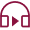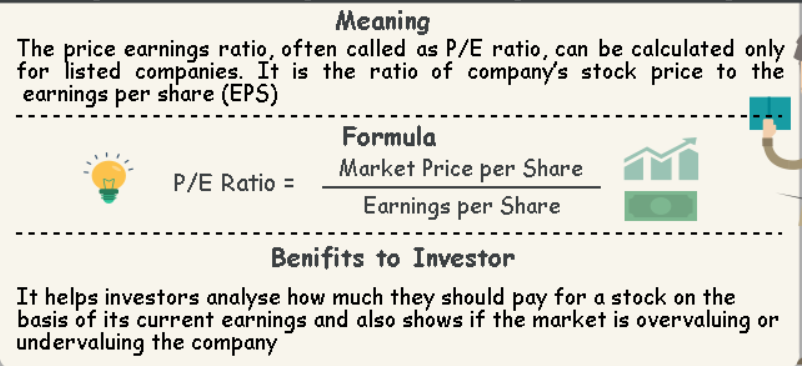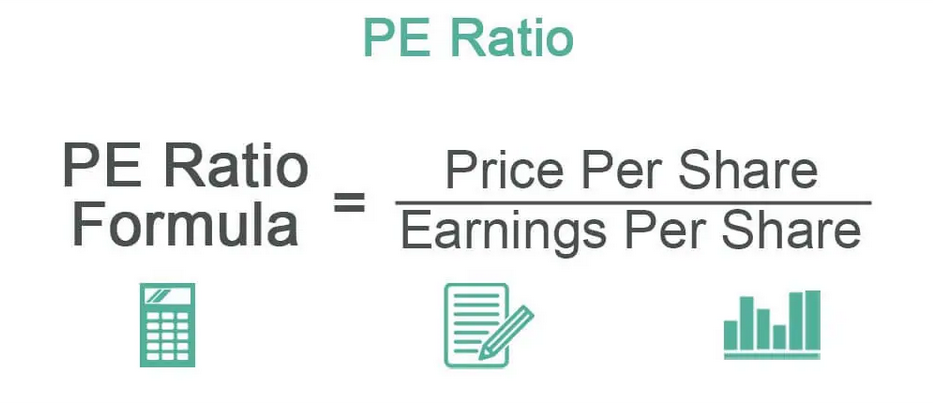Stock / Share Market
Last Updated: 2023-01-31T17:58:00+05:30## Price-to-Earnings Ratio (P/E ratio) Definition & Meaning

PE ratio stands for the price-earnings ratio. It is a valuation metric that provides investors with information about whether a company's shares are trading at an attractive price given their prospective earnings growth rate.

P/E ratio or price to earnings ratio is one of the most popular valuation tools. But what exactly is the PE ratio?

## What is the PE Ratio?

The price-earnings (PE) ratio is the most popular measure of stock-market valuation. It shows what you are paying for each dollar of earnings. If the PE ratio is high, then investors think that yields will be increased in future and vice versa.Basically, the P/E ratio is a measure of how much an investor is willing to pay for each rupee of earnings.

## Fundamentals of PE Ratio

P/E ratio is the price of a company's share divided by its earnings per share. The earnings, as the company reports them, are gross earnings.

Gross earnings is the value left over after all expenses, including taxes and interest on debt, have been paid. So-net profits are higher than gross profits. And net profits are higher than reported earnings.

But what is "net?" It is a lot less than gross. In some countries, like Japan, it can be a lot less. In most countries, the difference is not so extreme, but it is still there. So P/E ratios tend to be higher than they would be if we were using net earnings instead of reported earnings.

P/E ratio stands for the price to earnings ratio. Many people in the stock market use P/E because it shows how much you are paying for a dollar of profit.

## How is PE Ratio calculated?

The PE ratio is calculated by dividing the market price of a share by its earnings per share. The result is then multiplied by 100. A PE ratio of 8, for example, means that for every rupee of profit earned by the company, the shares are being sold at 8 rupees. A PE ratio of 15 means it's being sold at 15 rupees for every rupee of profit.

E.g., if the PE Ratio of TCS is ten, it means that the current market price of TCS is ten times its EPS.The PE ratio of a company tells us at what price investors are buying or selling the company's shares.

The higher the PE ratio of a company, the more expensive it is for investors to buy shares, so you can say that if the PE ratio of a company increases, then the value of its shares will also increase.

On the other hand, if the PE ratio of a company decreases, then its share value will also decrease because investors are finding it cheaper to purchase more shares, given that they are paying less money for each share.

## How to determine the PE Ratio?

There are two ways to find out what the PE ratio is.

You can look it up in a reference book, or you can do the math yourself.

The second method may not sound as convenient as the first, but it's easier than you might expect. It just requires that you know how to use three elementary formulas:

• one for calculating earnings per share (EPS),
• one for calculating the market price per share (market price divided by the total number of shares outstanding), and
• one for calculating PE ratio (market price divided by EPS).

The last formula is simply the reciprocal of the other two: 1 divided by market price divided by EPS.

### PE ratio = Price/Earnings

P/E ratio is a measure of a stock's market value relative to the net profit it generates.

If a company has a high P/E ratio, investors are willing to pay a significant price for each rupee of profit the company makes. For example, if XYZ stock has a P/E ratio of 100, it means that investors are willing to pay Rs.100 for every Rs.1 of profit made by XYZ.

If a company has a low P/E ratio, investors are not paying much for each rupee of profit made by the company. For example, if the P/E ratio of a company is 10, it means that investors are only willing to pay Rs.10 for every Rs.1 of profit made by the company.

## How to Use PE Ratios for Stock Market Investing?

The price-to-earnings ratio, or pe ratio, is the most common way to value a stock. It's also one of the concepts in finance that's hardest to understand.

There are pe ratios for individual companies and the market as a whole. The pe ratio for a company is usually written as price/earnings or p/e. For the market as a whole, it's written as price/earnings-to-growth, or p/eg (though you'll also see it written pe/e.g.).

It works by taking the company's stock price and dividing by its earnings per share (EPS), then multiplying by some growth rate. The result tells you how much you need to grow your earnings every year to justify the current stock price. If you don't think the company will grow that fast, you should wait until it does before buying its stock.

The best time to determine whether to buy or sell is when the stock gets close to its 52-week high.

The price/earnings ratio is an economic measure of the relationship between the price of a share of stock and the earnings per share. It is calculated by dividing the current market price of a share by its earnings per share.

## Understanding the PE Ratio

The most commonly used method to determine the fair market value of a share of common stock is to find its intrinsic value based on future cash inflows discounted at the risk-free rate. The resulting value is divided by the number of shares outstanding to get the fair market value per share (often called the intrinsic value).

The price/earnings ratio (or "P/E") is then determined by dividing this fair market value per share by either:

1) The company's actual earnings per share, or

2) The company's diluted earnings per share (if there are outstanding options and warrants).

## Why Do Investors Look at PE Ratios?

Investors look at PE Ratio while taking an investment decision. They invest in companies where earnings are growing faster than the stock price. In such a case, they believe that its earnings will ultimately justify its high price tag.

PE Ratio can be used to compare one company against another or even across sectors. Also, it helps in determining whether a company is undervalued or overvalued relative to its peers or underlying fundamentals.

It is easy to calculate how much money a company makes on each rupee invested by the shareholders through its Profit per Share (PPS) and further divide it by the current share price to develop a PE ratio for that company.

## Wrapping Up

PE Ratio is an important financial term. It denotes the relative "cheapness" or "expensiveness" of a company compared to its competitors. Diving into the numbers of a company represents some risk for making mistakes in a company's actual earnings. Understanding PE Ratio will facilitate your investment decisions.

Open Free Demat Account
Resend OTP